## Tuesday, 17 December 2013

### A Christmas Excel Challenge

Just a short, but seasonal, post this week - prompted by a quiz question my daughter was asked at school.

The question was, "How many gifts were received, in total, in the Christmas song The Twelve Days of Christmas?"

I thought that would make for an interesting Excel challenge.

So, the challenge is this:

Use Excel to answer the question, "How many gifts were received, in total, in the Christmas song The Twelve Days of Christmas?"

Explain in the comments how you would do this, remember though that it would be good practice to treat the 12 as a variable - so that you could enter any number of days of Christmas and the spreadsheet would calculate the total number of gifts.

In case you don't know the song, here are the lyrics:

On the first day of Christmas
my true love sent to me:
A Partridge in a Pear Tree

On the second day of Christmas
my true love sent to me:
Two Turtle Doves
and a Partridge in a Pear Tree

On the third day of Christmas
my true love sent to me:
Three French Hens
Two Turtle Doves
and a Partridge in a Pear Tree

On the fourth day of Christmas
my true love sent to me:
Four Calling Birds
Three French Hens
Two Turtle Doves
and a Partridge in a Pear Tree

On the fifth day of Christmas
my true love sent to me:
Five Golden Rings
Four Calling Birds
Three French Hens
Two Turtle Doves
and a Partridge in a Pear Tree

On the sixth day of Christmas
my true love sent to me:
Six Geese a Laying
Five Golden Rings
Four Calling Birds
Three French Hens
Two Turtle Doves
and a Partridge in a Pear Tree

On the seventh day of Christmas
my true love sent to me:
Seven Swans a Swimming
Six Geese a Laying
Five Golden Rings
Four Calling Birds
Three French Hens
Two Turtle Doves
and a Partridge in a Pear Tree

On the eighth day of Christmas
my true love sent to me:
Eight Maids a Milking
Seven Swans a Swimming
Six Geese a Laying
Five Golden Rings
Four Calling Birds
Three French Hens
Two Turtle Doves
and a Partridge in a Pear Tree

On the ninth day of Christmas
my true love sent to me:
Eight Maids a Milking
Seven Swans a Swimming
Six Geese a Laying
Five Golden Rings
Four Calling Birds
Three French Hens
Two Turtle Doves
and a Partridge in a Pear Tree

On the tenth day of Christmas
my true love sent to me:
Ten Lords a Leaping
Eight Maids a Milking
Seven Swans a Swimming
Six Geese a Laying
Five Golden Rings
Four Calling Birds
Three French Hens
Two Turtle Doves
and a Partridge in a Pear Tree

On the eleventh day of Christmas
my true love sent to me:
Eleven Pipers Piping
Ten Lords a Leaping
Eight Maids a Milking
Seven Swans a Swimming
Six Geese a Laying
Five Golden Rings
Four Calling Birds
Three French Hens
Two Turtle Doves
and a Partridge in a Pear Tree

On the twelfth day of Christmas
my true love sent to me:
12 Drummers Drumming
Eleven Pipers Piping
Ten Lords a Leaping
Eight Maids a Milking
Seven Swans a Swimming
Six Geese a Laying
Five Golden Rings
Four Calling Birds
Three French Hens
Two Turtle Doves
and a Partridge in a Pear Tree

Good luck. Post your approaches in the comments, and I will feature the slickest and/or the quirkiest solutions in next week's post, along with my own solution.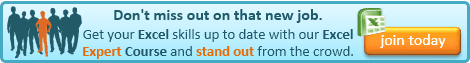If you enjoyed this post, go to the top of the blog, where you can subscribe for regular updates and get two freebies "The 5 Excel features that you NEED to know" and "30 Chants for Better Charts".

1.Hi all,

Cell A1 type some plain text like "How many days of Christmas"
Cell A2 type some plain text like "Number of gifts"

Cell B1 type the number of days. In this example 12
Cell B2 type the formula =(B1+1)/2*B1

1.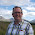Jan

Good start.

But that doesn't take account of the repeated gifts each day. e.g. you get a partridge on each of the 12 days, and two french hens one every day but the first, etc.

2.Hi Glen,

I missed that essence. Will share an update. Thanks

2.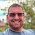A simple formula gives the amount of gifts per day as (n) therefore n (day number) =((+n*n)+n)/2 (day number squared, plus day number, and divide this all by two to get the amount of gifts

3.or if you mean the cumulative sum, then gifts =n*((n+1)*(n+2))/6 where n would be referenced to the day number (so 12 days gives 364 presents)

1.It is the cumulative sum that is required. I knew somebody would manage it in one formula - well done.

4.I just like the simplicity of numbering 1 to12 in row 1, 12 to1 in row 2, multiplying row 1 by row 2 in row 3 and summing the result of row three in the thirteenth column

1.Ray, that's a nice simple solution. More of an Excel solution than Simon's impressive mathematical one above.

5.The complexity I can see is that the partridge and the pear tree are two gifts, but I believe it is correct to treat each drummer and drum as one gift and each piper and pipe as other single gifts.

1.Correct, it's not a trick. :)

6.This comment has been removed by the author.

7.While it is not as elegant as Simon's mathmatical solution or Ray's helper row solution, how about a sumproduct solution?
=SUMPRODUCT((ROW(OFFSET(A1,0,0,NumDays)))*(NumDays-(ROW(OFFSET(A1,0,0,NumDays))-1)))
It simply multiplies the a list of row numbers from 1 to the number of days by a descending list of those same row numbers.

8.I like Ray’s simple solution. However to make it interactive with a variable number of days of Christmas I would put in A4 the number of days (you could even use a dropdown box to select 1 to 12) and link A2 to A4. In B2 I would put the following formula and copy it across =IF(A2>1,A2-1,0).

By selecting the number of days you will then get the total gifts.

9.Ok people, what about the geese a laying ... are they laying down or are they laying eggs which would be more gifts with each passing verse. Glen, you've finally found the magic potion to getting more commentators!!

1.ThIs little post does appear to have started something!

10.Though not as short as some others it does achieve the same answer (364 gifts) in one formula. Pasting the The Twelve Days of Christmas lyrics in excel and using the COUNTIF formula below:

=COUNTIF(range,"*Partridge*")*1+COUNTIF(range,"*Two*")*2+COUNTIF(range,"*Three*")*3+COUNTIF(range,"*Four*")*4+COUNTIF(range,"*Five*")*5+COUNTIF(range,"*Six*")*6+COUNTIF(range,"*Seven*")*7+COUNTIF(range,"*Eight*")*8+COUNTIF(range,"*Nine*")*9+COUNTIF(range,"*Ten*")*10+COUNTIF(range,"*Eleven*")*11+COUNTIF(range,"*Twelve*")*12

1.I like that. Certainly in the running for the quirkiest solution!

11.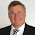err...so I did a spreadsheet and listed out how many presents each day. Total seems to be 364, which runs in a sequence over different presents 12,22,30,36,40,42,42,40,36,30,22,12 which is nicely symmetrical, but doesn't get you very far.
Then I realised that on each day where n is the number of the day my true love sends n+(n-1) presents. Then my arithmetic ran into the wall of maths and it's been so long. Can anyone tell me please if there is a way of applying a factor for the number of days in total to my little formula of n+(n-1) to give the correct solution for any number of days?

1.I got to the same point as you, trying to do it with one formula and my maths struggled at getting further than that. If you look at Simon Thacker's (2nd) comment above, he's done it.

2.He has indeed! very impressive. I'm now struggling with Why the +1 Why the +2 and why 6???

12.How about having a summary area to enter the variable day number and to return the result and then a separate table that populates based on the variable for the number of days that is entered...

A1 = "day"
A2 = enter the day number - the variable chosen can be e.g.12
B1 = "number of presents"
B2 =SUM(presents)

The formula in B2 should be entered once a table has been created and a range named as "presents"... see below.

Build the table... this assumes the partridge and the pear tree comprise 2 separate gifts - if they only represent one gift, remove the "+1" from the formulas in column E

D1 = "day number"
E1 = "# of presents"

D2 = 1
E2 =IF(D2="","",SUM(\$D\$2:D2)+1)
D3 =IFERROR(IF((D2+1)>\$A\$2,"",D2+1),"")
E3 =IF(D3="","",SUM(\$D\$2:D3)+1)

Copy D3 - E3 down to as many rows as desired - so if more verses are added, they will be accommodated.

Select the cells filled in columns D and E and click on insert table to convert this content to a table format.

Name column E "presents" and enter the formula in B2 = Sum(presents) using the "presents" named range in the formula.

Experiment with the variable - the number of days in A2 and see the result for # of presents update, and the table re-populate as appropriate.

13.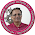Interesting challenge!!

14.I thought it would be fun to see if I could do this as a one cell array formula. Not the simplest method, I admit, but this works (don't forget to put Ctr;-Enter when you enter the formula):
{=2*(SUM(ROW(1:6)*(CHOOSE(ROW(1:6),12,11,10,9,8,7))))}
The maths is quite simple. We start off with 1*12, then add 1*12 plus 2*11, then add 1*12 plus 2*11 then 3*10, etc and end up with the whole series ending with 12*1. Therefore we have 12*1 once, but also 1*12 twelve times, 11*2 twice but 2*11 eleven times, which means that we have 2*((1*12)+(2*11)+(3*10)+(4*9)+(5*8)+(6*7))
This formula takes the values of rows 1-6 using the ROW function and multiplies them by the values 12 through 7 using the CHOOSE function, and multiplies the whole lot by 2 to get the answer of 364. Voila!

15.Another way of loking at it is this. On the first day, he gets 1 present, on the second 1+2, on the third 1+2+3 and so on. The formula for this as has been pointed out is n*(n+1)/2 for each day, which then needs to be added. So we can start with

=1+2*3/2+3*4/2+4*5/2+5*6/2+6*7/2+7*8/2+8*9/2+9*10/2+10*11/2+11*12/2+12*13/2

This can be simplified to

=1+(2*3+3*4+4*5+5*6+6*7+7*8+8*9+9*10+10*11+11*12+12*13)/2

and then we can develop a nice array formula, which is

{=1+SUM(ROW(2:12)*ROW(3:13)/2)}

Again, don't forget to hit Ctrl-Enter when entering the formula.

16.Glen:

Thanks for the nomination.

To clarify a few things when using the CONTIF formula. If your range includes the lyrics (example: On the fourth day of Christmas) excel will count fourth as Four. So either exclude in the range or use the formula below eliminating this extra count. The extra count occurs on; fourth, sixth, seventh, eighth, tenth & eleventh.

'=COUNTIF(\$D\$6:\$D\$118,"*Partridge*")*1+COUNTIF(\$D\$6:\$D\$118,"*Two Turtle*")*2+COUNTIF(\$D\$6:\$D\$118,"*Three French*")*3+COUNTIF(\$D\$6:\$D\$118,"*Four Calling*")*4+COUNTIF(\$D\$6:\$D\$118,"*Five Golden*")*5+COUNTIF(\$D\$6:\$D\$118,"*Six Geese*")*6+COUNTIF(\$D\$6:\$D\$118,"*Seven Swans*")*7+COUNTIF(\$D\$6:\$D\$118,"*Eight Maids*")*8+COUNTIF(\$D\$6:\$D\$118,"*Nine Ladies*")*9+COUNTIF(\$D\$6:\$D\$118,"*Ten Lords*")*10+COUNTIF(\$D\$6:\$D\$118,"*Eleven Pipers*")*11+COUNTIF(\$D\$6:\$D\$118,"*Twelve*")*12

Happy Holidays

17.Sorry, I left out Drummers on Twelve.

Now that I think about it, don't use the numeral words, just use the gift name, duh

That's enough fun for one week. Thanks Glen.

18.I made mine anticipating pedants and with the option for expansion (never a wrong time for best practice!).

So, six rows in my main table:
Gift - free text eg "Doves", "Geese"
Day - starting at 0 to help make copying down formulae easy and going down to 12
New items from previous days - this is the fresh batch of gifts which are duplicates of yesterdays, so simply the previous value in this column plus the previous value from the New. To keep the formula working need to hard code a 0 for this on day 0.
Carried forward from previous days - All the gifts we've had up to this point. So the previous day's values for New, New items from previous days and this column.
Total - The total number of gifts that day. So the sum of New, New items from previous days and carried forward from previous days for that day.

Assuming that:
the a goose is one gift and the egg it is a-laying is another gift
each drummer has additional gifts of 1 x drum 2 x drumsticks
each piper has a pipe as an additional gift
each maid is delivered with a cow
each partridge comes with a tree

we have 425 gifts

If you replicate this, don't forget to sort your lookup table for vlookup.

~Robskii the Red Nosed Rer

19.Simple & Sily solution is count Paragraphs.....e.g. Para 1 = Gift 1; Para 2 = 2+1; Para 3 = 3+2+1; Para 4 = 4+3+2+1.....Para 12 = 12+11+10....+1 = 364......No advance excel tricks required

20.To All:

To add a twist to this fun, use this VBA code, add your button to your worksheet, assign the Macro name and save file as xlsm.

Now highlight a range of "text" like the The Twelve Days of Christmas, click on the button, type in the word or words to count. Example: Five Golden Rings = 8

Code:
Dim Count As Integer
Dim Target As String
Dim Cell As Object
Dim N As Integer

Sub Target_Count()
Count = 0
Target = InputBox("character(s) to find?")
If Target = "" Then GoTo Done
For Each Cell In Selection
N = InStr(1, cell.Value, target)
While N <> 0
Count = count + 1
N = InStr(n + 1, cell.Value, target)
Wend
Next Cell
MsgBox count & " Occurrences of " & target
Done:
End Sub

1.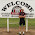A solution, to show a VBA Function call itself recursively:

Option Explicit

Const nrofdays As Integer = 12
Dim nrofgifts As Integer